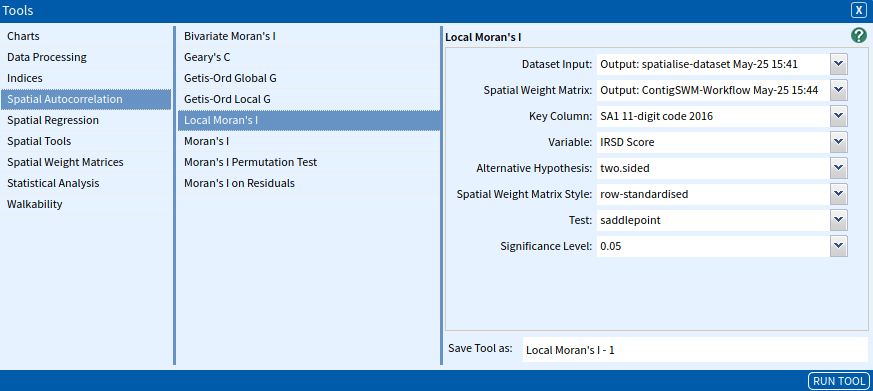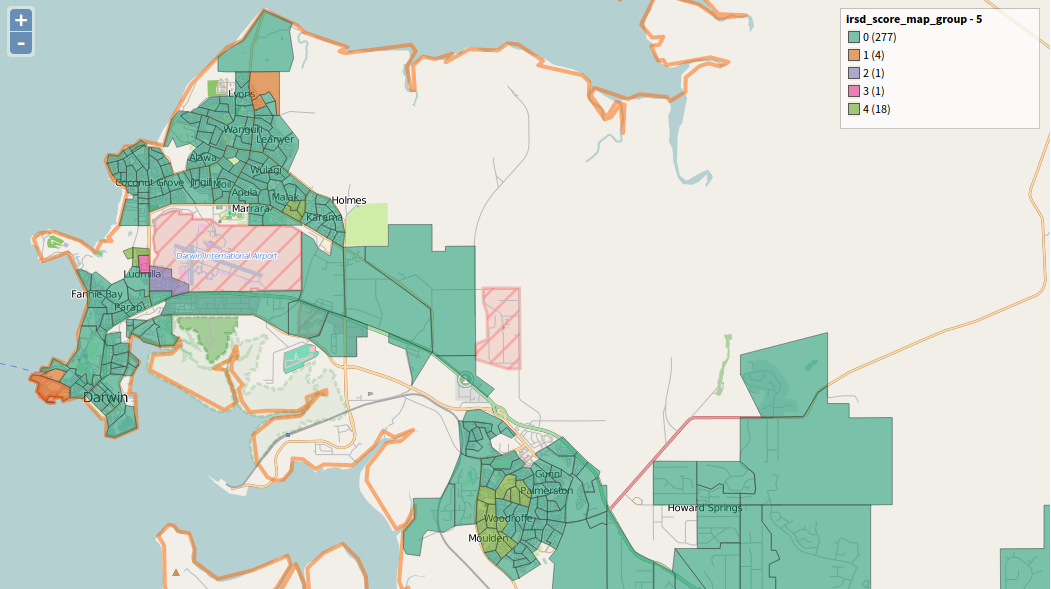Local Moran's I

Local Moran’s I is a local spatial autocorrelation statistic that identifies local clusters or local outliers to understand their contribution to the ‘global’ clustering statistic. It was developed by Anselin (1995) as a class of local indicators called Local Indicators of Spatial Association (LISAs). The local Moran’s I statistic offers insight into the behaviour of data at local levels, by providing a decomposition of the Moran’s I global statistic into the degree of spatial association associated with each observation. LISAs serve two purposes in exploratory spatial data analysis (ESDA): they indicate local spatial clusters and they perform sensitivity analysis (identify outliers).

Anselin (1995, p. 94) defines LISA as:

a. the LISA for each observation gives an indication of the extent of significant spatial clustering of similar values around each observation; and
b. the sum of LISAs for all observations is proportional to a global indicator of spatial association.

As with global measures, LISAs test whether the observed spatial pattern of a variable of interest amongst areas is extreme or is likely or expected, given a random geographic distribution of the variable.

Local Moran’s I is calculated as:

$\LARGE{I_i=z_i\sum_{j}w_{ij}z_j}$

Where the $z_i$ and $z_j$ are the observations in deviations from the mean and $w_{ij}$ is the spatial weight matrix element. Positive values of $I_i$ suggest that there is a spatial cluster of similar values and negative values represent a spatial cluster of dissimilar values.

Significance testing of the local Moran statistics can be somewhat problematic. Unlike the $G_i$ and $G_i*$ statistics, the local Moran does not conform to a common distribution and so the test under a normality assumption should be treated with caution. Instead Anselin (1995) suggests a conditional randomisation or permutation approach to give so-called pseudo significance levels. Tiefelsdorf & Boots (1998) published the exact reference distribution of Moran’s I, but in a later paper recommends an application of the Saddlepoint approximation, as it “outperforms other approximation methods with respect to its accuracy and computational costs” (Tiefelsdorf, 2002, p. 187). The Local Moran’s I tool in the AURIN Portal provides results for a normal distribution, a Saddlepoint approximation of the standard deviate and the exact standard deviate.

SET UP

To demonstrate this tool in use, we will look at socio-economic disadvantage data in Greater Darwin to examine the extent of spatial-autocorrelation.

Select Greater Darwin GCCSA as your area.

Select ABS – Socio-Economic Indexes for Areas (SEIFA) – The Index of Relative Socio-economic Disadvantage (SA1) 2016 as your dataset, select IRSD Score as the variable.

Use the Spatialise Aggregated Dataset tool to Spatialise the dataset.

Use the Contiguous Spatial Weight Matrix tool to build a Spatial Weights Matrix for the spatialised dataset, using 1st order, row-standardised, Queen contiguity.

Inputs

Open the Local Moran’s I tool (Tools → Spatial Autocorrelation → Local Moran’s I) and enter the following parameters:

• Dataset Input: The dataset that contains the variable to be tested. Select the Spatialised Dataset.
• Spatial Weights Matrix: The spatial weight matrix to be used. Select the Contiguous Spatial Weight Matrix.
• Key Column: Specify the unique codes for your areas. Select SA1 11-digit Code.
• Variable: The variable to be tested. Select IRSD Score.
• Alternative Hypothesis: Specifies the alternative hypothesis. Select two.sided.
• two.sided: a priori assumption that the difference between Ii and the expected E[Ii] is not equal to zero (spatial autocorrelation).
• greater: a priori assumption that Ii is greater than the expected E[Ii] (positive spatial autocorrelation).
• less: a priori assumption that Ii is less than the expected E[Ii] (negative spatial autocorrelation).
• Spatial Weights Matrix Style: The standardisation style used for the input spatial weight matrix. Select row.standardised.
• Test: Indicates the shape of the distribution used to test for significance. Select saddlepoint.
• exact: Implements the Tiefelsdorf & Boots (1998) application of the exact reference distribution.
• Significance Level: Set the level of significance for your test. Select 0.05.

The input parameters are summarised in the image below, once complete click Run Tool.Outputs

Your output will be a dataset that can be mapped based on a number of the variables produced by the analysis. These are explained below:

• li: The Local Moran’s I statistic.
• Saddlepoint: The standard deviate of the Saddlepoint Local Moran’s I statistic, this can also be interpreted as a Z-score (only output if “saddlepoint ” is selected).
• Exact SD: The standard deviate of the Exact Local Moran’s I statistic, this can also be interpreted as a Z-score (only output if “exact” is selected).
• Pr_Sad: The p-value of the saddlepoint Local Moran’s I statistic (only output if “saddlepoint” is selected).
• Pr_Exact: The p-value of the exact Local Moran’s I statistic (only output if “exact” is selected).
• <input_variable>_Lagged: the average value of the variable for the areas surrounding each area.
• <input_variable>_Scaled: the value of the variable for your area scaled (z-score).
• <input_variable>_Lagged_Scaled: the average value of the variable for the areas surrounding each area, scaled (z-score).
• <input_variable>_map_group: the number representing the group that your area belongs to: 0 = Not Significant, 1 = High surrounded by High; 2 = High surrounded by Low; 3 = Low surrounded by High; and 4 = Low surrounded by low.
• <input_variable>_map_group_name: the names of the above groups.

You can create a visualisation using the Choropleth tool on one of the above variables. For the image below, we have chosen to use the variable irsd_score_map_group_name. The tool produces five groups in this variable, listed above. Using a Qualitative and Dark2 palette:

• Orange indicates areas of high IRSD index scores surrounded by other high scores (low disadvantage),
• Purple indicates high scores surrounded by low scores,
• Pink indicates low scores surrounded by high scores,
• Light-green indicates low areas surrounded by low scores, and
• Dark-green represents areas with no statistical significance.Anselin, L. (1995). Local Indicators of Spatial Association – LISA. Geographical Analysis27(2), 93-115.

Tiefelsdorf, M. & Boots, B. (1995). The Exact Distribution of Moran’s I. Environment and Planning A: Economy and Space. 27(6), 985-999.

Tiefelsdorf, M. (2002). The Saddlepoint Approximation of Moran’s I’s and Local Moran’s I’s Reference Distributions and Their Numerical Evaluation. Geographical Analysis. 34(3), 187-206.

Looking for Spatial Data?

You can browse the AURIN Data Discovery:How can you Create Impact?